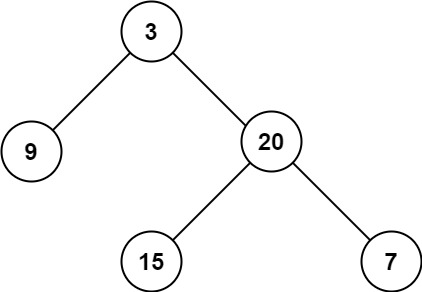Coding

# LeetCode Maximum Depth of Binary Tree Solution

0
(0)

Given the `root` of a binary tree, return its maximum depth.

A binary tree’s maximum depth is the number of nodes along the longest path from the root node down to the farthest leaf node.

Example 1:```Input: root = [3,9,20,null,null,15,7]
Output: 3
```

Example 2:

```Input: root = [1,null,2]
Output: 2
```

Constraints:

• The number of nodes in the tree is in the range `[0, 104]`.
• `-100 <= Node.val <= 100`

Solution

Maximum Depth of binary tree can be thought as same as height of binary tree. You can think of height as number of nodes from root to farthest leaf (with both root and leaf included).

Once you understand the problem, as I have done with other binary tree problems, I’ll give recursion a try. So height of a binary tree can be maximum of height of left sub tree and right sub tree and we need to add one to it to account for parent/root node.

Below is the Java code for this.

`````` public int maxDepth(TreeNode root) {
if (root == null) return 0;
else return Math.max(maxDepth(root.left), maxDepth(root.right)) + 1;
}``````

Pretty sleek right. 🙂

Let’s check time and space complexity of it.Pretty fast as well. 🙂 There is an iterative solution as well but it is not as intuitive as the above recursive approach.

Click on a star to rate it!

Average rating 0 / 5. Vote count: 0

No votes so far! Be the first to rate this post.

As you found this post useful...

We are sorry that this post was not useful for you!

Let us improve this post!

Tell us how we can improve this post?